Geometry

# Triangles - Compare Similar Triangles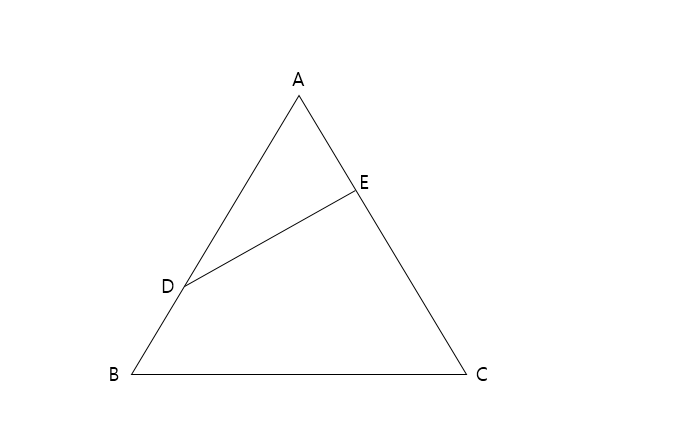In the above triangle, point D divides $$\overline{AB}$$ in the ratio $$\ 2:1$$ and point E divides $$\overline{AC}$$ in the ratio $$\ 1:2.$$ If the area of $$\triangle ABC$$ is $$23,$$ what is the area of $$\triangle ADE?$$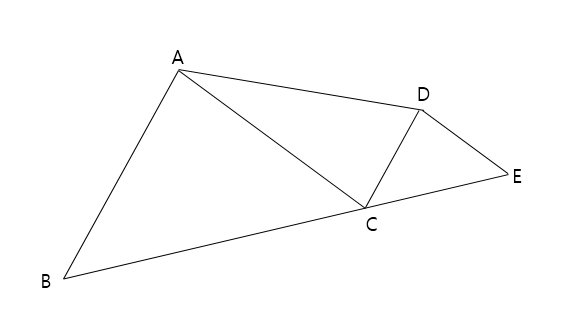Triangle $$\triangle ABC$$ is similar to $$\triangle DCE$$ with $$\overline{AB} \parallel \overline{CD}.$$ If the area of $$\triangle ABC$$ is $$36$$ and the area of $$\triangle DCE$$ is $$9,$$ what is the area of $$\triangle ACD?$$

Note: The above diagram is not drawn to scale.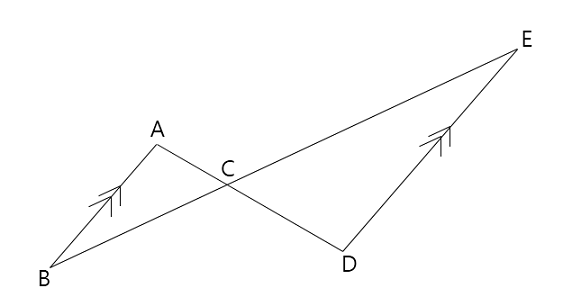$$\overline{AB}$$ is parallel to $$\overline{DE}$$, the length of $$\overline{AC}$$ is $$3,$$ and the length of $$\overline{CD}$$ is $$11.$$ If the area of $$\triangle ABC$$ is $$4,$$ what is the area of $$\triangle CDE$$ ?

Note: The above diagram is not drawn to scale.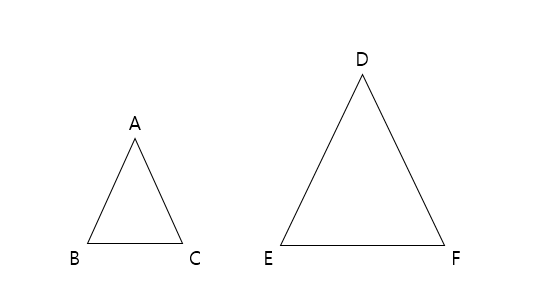Triangle $$\triangle ABC$$ is similar to $$\triangle DEF$$, and the ratio of their areas is $$9:25.$$ If the length of $$\overline{DE}$$ is $$70,$$ what is the length of $$\overline{AB}$$?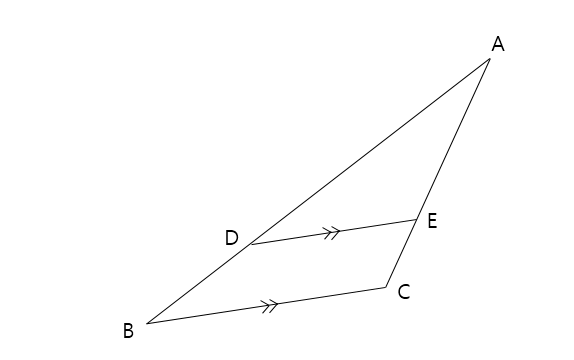$$\overline{BC}$$ is parallel to $$\overline{DE}$$, the length of $$\overline{AD}$$ is $$7,$$ and the length of $$\overline{BD}$$ is $$3.$$ If the area of $$\triangle ABC$$ is $$17,$$ what is the area of $$\triangle ADE$$ ?

Note: The above diagram is not drawn to scale.

×